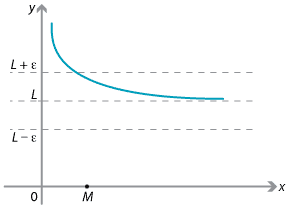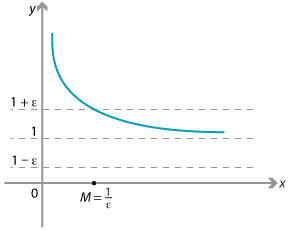### Formal definition of a limit

In this module, the notion of limit has been discussed in a fairly informal manner. To be able to prove results about limits and capture the concept logically, we need a formal definition of what we mean by a limit. We will only look here at the precise meaning of $$\lim\limits_{x\to \infty} f(x) = L$$, but there is a similar definition for the limit at a point.

In words, the statement $$\lim\limits_{x\to \infty} f(x) = L$$ says that $$f(x)$$ gets (and stays) as close as we please to $$L$$, provided we take sufficiently large $$x$$. We now try to pin down this notion of closeness.

Another way of expressing the statement above is that, if we are given any small positive number $$\varepsilon$$ (the Greek letter epsilon), then the distance between $$f(x)$$ and $$L$$ is less than $$\varepsilon$$ provided we make $$x$$ large enough. We can use absolute value to measure the distance between $$f(x)$$ and $$L$$ as $$|f(x) - L|$$.

How large does $$x$$ have to be? Well, that depends on how small $$\varepsilon$$ is.

The formal definition of $$\lim\limits_{x\to \infty} f(x) = L$$ is that, given any $$\varepsilon > 0$$, there is a number $$M$$ such that, if we take $$x$$ to be larger than $$M$$, then the distance $$|f(x) - L|$$ is less than $$\varepsilon$$.The value of $$f(x)$$ stays within $$\varepsilon$$ of $$L$$ from the point $$x = M$$ onwards.

For example, consider the function $$f(x) = \dfrac{x+1}{x}$$. We know from our basic work on limits that $$\displaystyle \lim_{x\to \infty} f(x) = 1$$. For $$x > 0$$, the distance is

$\bigl|f(x) - 1\bigr| = \Bigl|\dfrac{x+1}{x} - 1\Bigr| = \dfrac{1}{x}.$

So, given any positive real number $$\varepsilon$$, we need to find a real number $$M$$ such that, if $$x>M$$, then $$\dfrac{1}{x} < \varepsilon$$. For $$x>0$$, this inequality can be rearranged to give $$x > \dfrac{1}{\varepsilon}$$. Hence we can choose $$M$$ to be $$\dfrac{1}{\varepsilon}$$.Exercise 8

Let $$f(x) = \dfrac{2x^2+3}{x^2}$$. Given $$\varepsilon > 0$$, find $$M$$ such that if $$x>M$$ we have $$|f(x)-2|< \varepsilon$$. Conclude that $$f(x)$$ has a limit of 2 as $$x\to \infty$$.

While the formal definition can be difficult to apply in some instances, it does give a very precise framework in which mathematicians can properly analyse limits and be certain about what they are doing.

Next page - Links forward - The pinching theorem